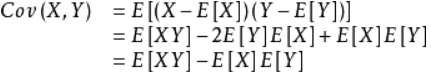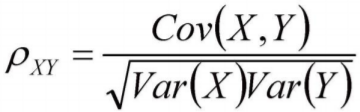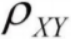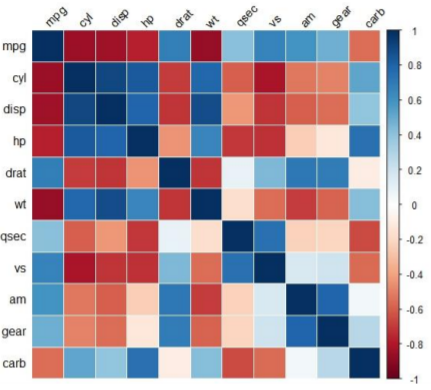协方差，协方差矩阵，相关系数

1.协方差

X,Y为两个随机变量，则它们的协方差值为：Cov(X,Y) = E(XY+E[X]E[Y]-E[X]Y-E[Y]X)

=E(XY)+E(E[X]E[Y])-E(E[X]Y)-E(E[Y]X)

=E(XY)+E[X]E[Y]-E[X]E[Y]-E[X]E[Y]

= E[XY] - E[X]E[Y]

如果两个变量的协方差为正，说明它们的变化方向相同

如果两个变量的协方差为负，说明它们的变化方向相反

如果两个变量的协方差为0，说明两个变量不相关

2.协方差矩阵

假如有X1，X2，X3，...Xn一组变量，

4.相关系数

协方差的上界：

有X，Y变量，直观的我们可以理解，变量X和自己本身的协方差肯定要大于等于变量X和Y的协方差，因为变量X和自己本身的变化趋势是完全一样的，所以给出下面的式子：var(X)代表X变量的方差

cov(X,Y) = |E(XY) - E(X)E(Y)| <=cov(X,X) = E(X^2) - E(X)*E(X)=var(X)=delta1^2    记为T1

cov(X,Y) = |E(XY) - E(X)E(Y)| <=cov(Y,Y) = E(Y^2) - E(Y)*E(Y)=var(Y)=delat2^2     记为T2

cov(X,Y) <=delta1*delta2，delta就是方差开方得到的<= 1

4.相关系数矩阵